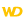HelpWLanguageWLanguage functionsStandard functionsNumeric values management functionsMathematical functionsPowerPresentationExampleEquivalenceSee alsoAbsArcCosArcSinArcTan2ArcTanCoTanConversionCosDecimalPartDecimalToSexagesimalExpFactorialIntegerPartIsEvenIsOddLnLogMath functionsPowerRootRoundRoundCeilRoundFloorRoundToMultipleSexagesimalToDecimalSinTangent
 Equivalence WINDEVWEBDEVWINDEV MobileOthersPower (Function) In french: Puissance Raise a number to a power.Example // 8 to the power of 5ResPower = Power(8, 5)// Returns 32768 Syntax = Power( , ) : RealResult of to the power of . : Integer or realNumber that will be raised to the power. : Integer or realExponent. RemarksEquivalencePower is equivalent to the ^ arithmetic operator. The syntax of this operator is as follows: ^ Component: wd280mat.dll Minimum version required Version 9 This page is also available for…